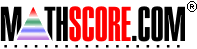Math Practice Online > free > lessons > Florida > 6th grade > Order Decimals

## Order Decimals

Practice o rdering decimals from least to greatest or greatest to least.

 Sample Problems for Order Decimals Lesson for Order Decimals

### This topic aligns to the following state standards

Grade 4: Num 3. Compares and orders commonly used fractions and decimals to hundredths using concrete materials, drawings, and numerals.
Grade 5: Num 3. Compares and orders commonly used fractions, percents, and decimals to thousandths using concrete materials, number lines, drawings, and numerals.
Grade 6: Num 1. Compares and orders fractions and decimals using graphic models, number lines, and symbols.
Grade 6: Num 2. Compares and orders fractions, decimals, and common percents.
Grade 7: Num 1. Compares and orders integers, fractions, decimals, numbers with exponents, and numbers expressed as percents or in scientific notation, including ordering on a number line.
Grade 8: Num 1. Compares and orders fractions, decimals, integers, and radicals using graphic models, number lines, and symbols.
Grade 8: Num 2. Compares and orders numbers expressed in absolute value, scientific notation, integers, percents, numbers with exponents, fractions, decimals, radicals, and ratios.
Grade 9: Num 2. understands the relative size of integers, rational numbers, irrational numbers, and real numbers

Copyright Accurate Learning Systems Corporation 2008.